### Home > PC > Chapter 12 > Lesson 12.1.1 > Problem12-6

12-6.
1. Let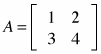and let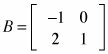. Homework Help ✎

1. Find AB and BA.

2. What can you conclude about multiplying matrices?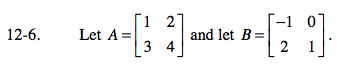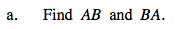When multiplying matrices, multiply the rows of the first matrix by the columns of the second matrix.

Now simplify and then calculate BA on your own.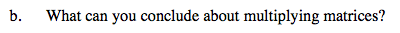Does AB = BA?